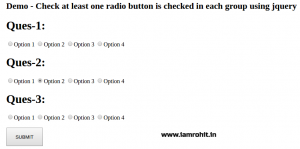# How to check at least one radio button is checked in each group using jquery

If you have created any question and answer system or polling system in website then you have to validate form to check at least one radio button is checked in each question and answer group. You can easily validate question group by Using jQuery if radio is checked. as same you can validate if one radio button in each group is Checked. In Following example I have created three demo question with 4 option each and we need to validate form before submitting that at one radio button is checked in each group.### Checking at least one radio button is checked in each group using jquery

#### HTML

Following are the demo question and answer, each question with 4 answer and we need to validate at least one radio button is checked in each question group.

```<h1>Ques-1:</h1> <input type="radio" name="ques1" value="1" />Option 1 <input type="radio" name="ques1" value="2" />Option 2 <input type="radio" name="ques1" value="3" />Option 3 <input type="radio" name="ques1" value="4" />Option 4   <h1>Ques-2:</h1> <input type="radio" name="ques2" value="1" />Option 1 <input type="radio" name="ques2" value="2" />Option 2 <input type="radio" name="ques2" value="3" />Option 3 <input type="radio" name="ques2" value="4" />Option 4   <h1>Ques-3:</h1> <input type="radio" name="ques3" value="1" />Option 1 <input type="radio" name="ques3" value="2" />Option 2 <input type="radio" name="ques3" value="3" />Option 3 <input type="radio" name="ques3" value="4" />Option 4   <br/><br/> <button type="submit" style="height:50;width:100">SUBMIT</button>```

#### Javascript

Add below script, On submit button click to validate form before submitting at least one radio button is checked in each group using jquery, don’t forget to add jquery library on page.

```<script src="https://ajax.googleapis.com/ajax/libs/jquery/3.2.1/jquery.min.js"></script> <script> \$(function() { \$("button[type='submit']").click(function(){ var check = true; \$("input:radio").each(function(){ var name = \$(this).attr("name"); if(\$("input:radio[name="+name+"]:checked").length == 0){ check = false; } });   if(check){ alert('HURREY!! One answer in each question is checked.'); }else{ alert('Please select at least one answer in each question.'); } }); }); </script>```